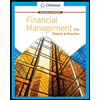# Compute the price of a 5.9 percent coupon bond with 15 years left to maturity and a market interest rate of 9.6 percent. (Assume interest payments are semiannual.) (Do not round intermediate calculations. Round your final answer to 2 decimal places.)   Is this a discount or premium bond? premium bond discount bond

Question

Compute the price of a 5.9 percent coupon bond with 15 years left to maturity and a market interest rate of 9.6 percent. (Assume interest payments are semiannual.) (Do not round intermediate calculations. Round your final answer to 2 decimal places.)

Is this a discount or premium bond?

• discount bond

Expert Solution

### Want to see the full answer?

Check out a sample Q&A hereStudents who’ve seen this question also like:Financial Management: Theory & Practice
16th Edition
ISBN: 9781337909730
Author: Brigham
Publisher: Cengage